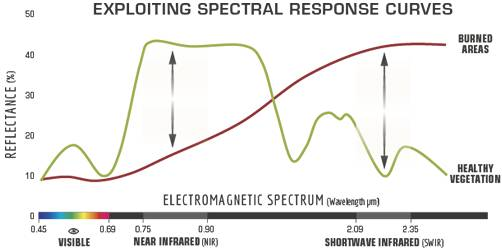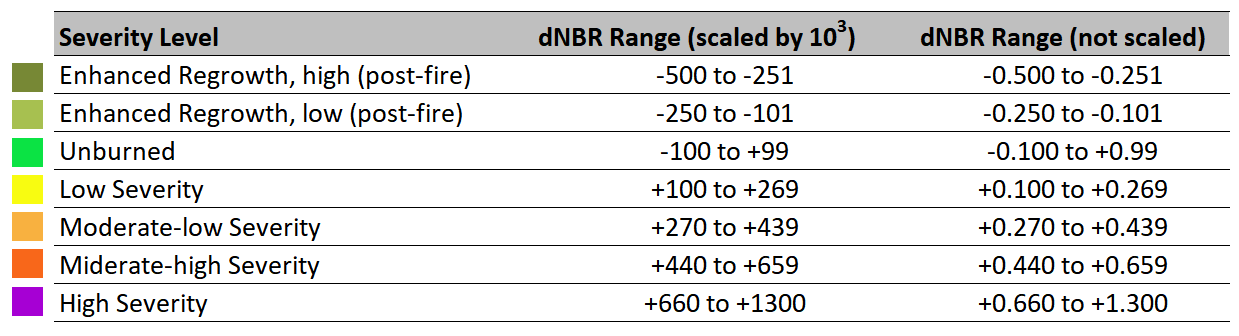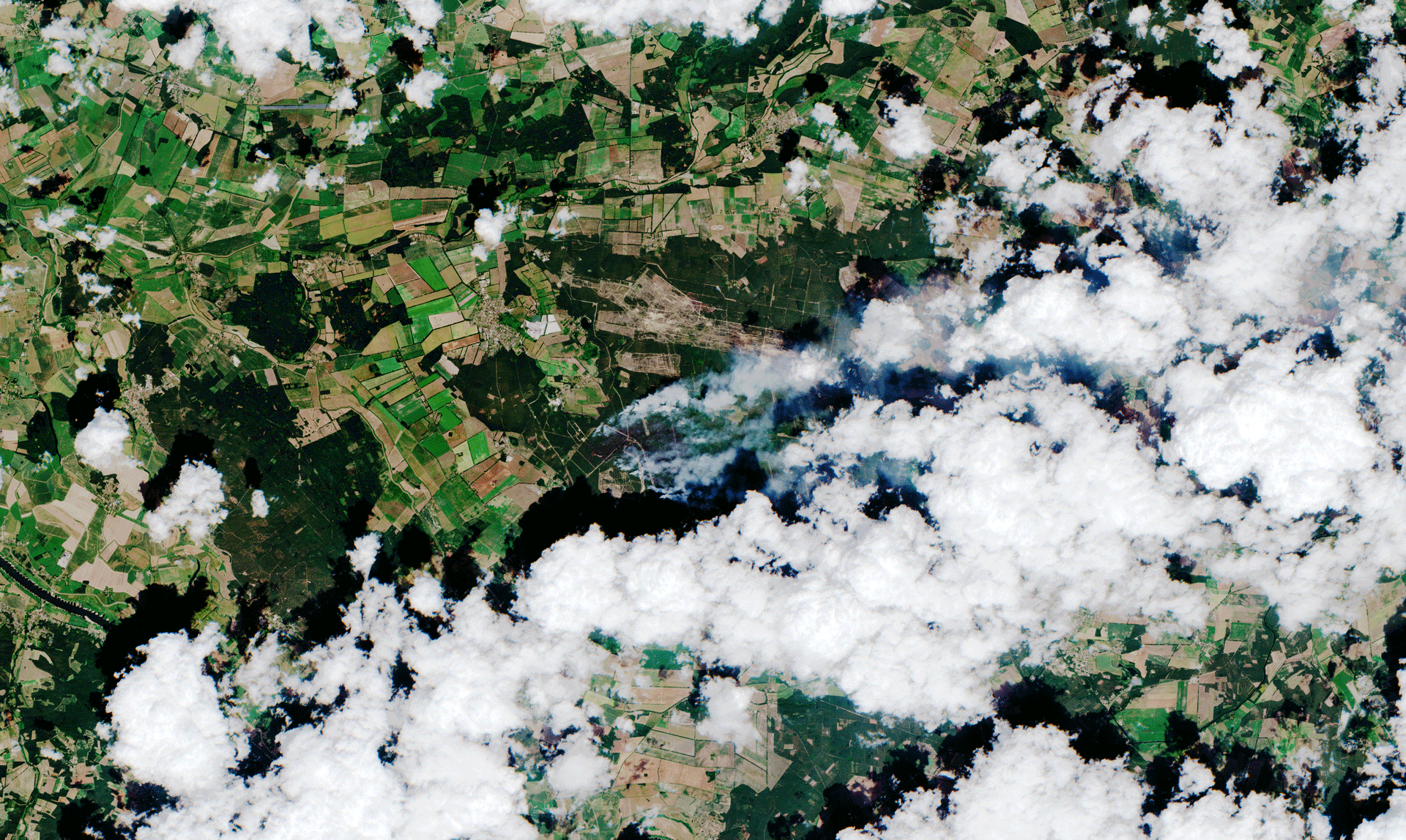# Burn Area Mapping¶

This notebook contains the calculations necessary for mapping the burn severity of the previously selected products. This burn severity is measured as the Normalized Burn Ratio (NBR). The development of this burn ratio over time is described using the $${\Delta}$$NBR (or dNBR), which is defined as:

${\Delta}\text{NBR} = \text{NBR}_\text{pre-fire} - \text{NBR}_\text{post-fire}$

Instead of only calculating values by overlaying different bands in one product, the $${\Delta}$$NBR adds a time dimension to determine changes that have occurred in the time span between the two chosen products.

## Methodology¶

The NBR of the products downloaded in the previous notebook is calculated using the notebook Generalized Spectral Index Calculation. These NBR values are plotted side-by-side to get a visual impression of the changes that may have occurred between two given dates. The $${\Delta}$$NBR is calculated as described by the RUS Material and, if showing signs of burned areas, compared to data given by the responsible German ministries.

## Calculcations¶

### Setup¶

import geopandas as gpd
from pathlib import Path
from sentinel_helpers import geodataframe_on_map

nbr_path = Path('resources/spectral_indices/')
product_path = Path('resources/forest_fires/')


### Treuenbrietzen 2018¶

The first case is the wildfire in August 2018 that led to the evacuation of three villages (source). The following files contain their respective OpenStreetMap geometries and pre-calculated NBR values for the area:

treuenbrietzen_nbr_2018 = sorted(nbr_path.glob('*2018*NBR*.tif'))
treuenbrietzen_ndwi_2018 = sorted(nbr_path.glob('*2018*NDWI*.tif'))
treuenbrietzen_nbr_2018

[PosixPath('resources/spectral_indices/T33UUT_20180822T101019_NBR_10m.tif'),
PosixPath('resources/spectral_indices/T33UUT_20180906T101021_NBR_10m.tif'),
PosixPath('resources/spectral_indices/T33UUT_20180919T102021_NBR_10m.tif')]


The treuenbrietzen_geom is a collection of three points:

treuenbrietzen_geom = gpd.read_file(product_path / 'evacuated_2018.json')
# geodataframe_on_map(treuenbrietzen_geom)
treuenbrietzen_geom

place_id osm_type osm_id display_name place_rank category type importance icon geometry
0 3285415 node 387079362 Frohnsdorf, Treuenbrietzen, Potsdam-Mittelmark... 19 place village 0.495 https://nominatim.openstreetmap.org/ui/mapicon... POINT (12.90217 52.05466)
1 554316 node 226935349 Klausdorf, Treuenbrietzen, Potsdam-Mittelmark,... 19 place village 0.495 https://nominatim.openstreetmap.org/ui/mapicon... POINT (12.94213 52.04879)
2 303964452 node 282202396 Tiefenbrunnen, Treuenbrietzen, Potsdam-Mittelm... 22 place isolated_dwelling 0.420 None POINT (12.94439 52.03532)

#### NBR Plots¶

The NBR values for all dates are shown side by side to get a first visual impression of the changes that have occurred.

import numpy as np
import matplotlib.pyplot as plt
from sentinel_helpers import scihub_band_paths, scihub_band_date, RasterReaderList
import rasterio as r
import rasterio.plot as rplt

# we can save some time by reading only parts of the product we are interested in
from rasterio.features import geometry_window

def plot_nbrs(products, geom):
fig, axes = plt.subplots(nrows=1, ncols=3, figsize=(18,18))

# we need to reproject from WGS84 so the geometry can be correctly plotted on the map

# we don't need the entire NBR, we only use a slice

# ensure that subplot colors are chosen on a fixed scale
vmin = nbrs.min()
vmax = nbrs.max()

ax.tick_params(axis='x', labelrotation=90)
rplt.show(nbr,
ax=ax,
transform=window_transform,
cmap='Greys',
title=product_dt.strftime('%Y-%m-%d'),
vmin=vmin,
vmax=vmax)
_geom.plot(ax=ax, facecolor='none', edgecolor='red')

# increase horizontal whitespace between subplots

# add colorbar using the last image
img = axes[-1].get_images()
fig.colorbar(img, ax=axes, shrink=0.2)


Red dots mark the location of the evacuated villages, the background shows the calculated NBR values, where areas with a low NBR value are brighter and areas with a high NBR value are darker.

plot_nbrs(treuenbrietzen_nbr_2018, treuenbrietzen_geom)The image before immediately the fire shows no mark, but the plots for September 6 and September 19 both show an area with very low NBR values that appeared after the fire started.

The NBR can be interpreted as follows:

Healthy vegetation shows a very high reflectance in the NIR1, and low reflectance in the SWIR2 portion of the spectrum - the opposite of what is seen in areas devastated by fire. Recently burnt areas demonstrate low reflectance in the NIR and high reflectance in the SWIR, i.e. the difference between the spectral responses of healthy vegetation and burnt areas reach their peak in the NIR and the SWIR regions of the spectrum.Figure 2. Comparison of the spectral response of healthy vegetation and burned areas. Source: U.S. Forest service.

To benefit from the magnitude of spectral difference, NBR uses the ratio between NIR and SWIR bands, according to the formula shown below. A high NBR value indicates healthy vegetation while a low value indicates bare ground and recently burnt areas. Non-burnt areas are normally attributed to values close to zero.

$$\text{NBR} = \frac{\text{NIR} - \text{SWIR}}{\text{NIR} + \text{SWIR}}$$

Source: UN-Spider Knowledge Portal

#### dNBR Calculation¶

In order to calculate $${\Delta}$$NBR, the NBR after the fire is sutracted from the NBR before the fire. Reminder:

${\Delta}\text{NBR} = \text{NBR}_\text{pre} - \text{NBR}_\text{post}$

As suggested in the RUS Training Material, the NBR is masked where surface pixels probably represent water (NDWI >= 0). This avoids false positives.

def water_mask(pre_ndwi, post_ndwi, window):
return (pre.data >= 0) | pre.mask | (post.data >= 0) | post.mask

def calculate_dnbr(pre_nbr, post_nbr, pre_ndwi, post_ndwi, geom=[]):
if len(geom):
# if a geometry is passed, perform all calculations only in the
# surroundings if this geometry
else:
window = window_transform = None

# we need to mask invalid pixels in any of the input files for the resulting file
dnbr = pre_fire - post_fire

return (dnbr, window, window_transform)


dNBR values can vary from case to case, and so, if possible, interpretation in specific instances should also be carried out through field assessment; in order to obtain the best results. However, the United States Geological Survey (USGS) proposed a classification table to interpret the burn severity, which can be seen below (Table 1).Table 1. Burn severity levels obtained calculating dNBR, proposed by USGS.

Source: UN-Spider Knowledge Portal

A custom color scheme for matplotlib is constructed to match the values given in the table above. This is used to define a function that plots $$\Delta$$NBR values using different color scales:

from matplotlib.colors import BoundaryNorm, ListedColormap

# define discrete color scale based on table above
dnbr_cmap = ListedColormap(['#778835', '#a7c050', '#0be344', '#f8fc11', '#f8b140', '#f8671a', '#a600d4'])
boundaries = [-0.5, -0.25, -0.1, 0.1, 0.27, 0.44, 0.66, 1.3]
dnbr_norm = BoundaryNorm(boundaries, dnbr_cmap.N, clip=True)

def plot_dnbr(dnbr, dnbr_crs, dnbr_transform, geom):
fig, (ax1, ax2) = plt.subplots(ncols=2, figsize=(16, 8))
_geom = geom.to_crs(dnbr_crs)

# plot black and white image
rplt.show(dnbr, ax=ax1, transform=dnbr_transform, cmap='Greys', vmin=boundaries, vmax=boundaries[-1])
ax1.tick_params(axis='x', labelrotation=90)
_geom.plot(ax=ax1, facecolor='none', edgecolor='red')

# plot image using colormap from above
rplt.show(dnbr, ax=ax2, transform=dnbr_transform, cmap=dnbr_cmap, norm=dnbr_norm)
ax2.tick_params(axis='x', labelrotation=90)
_geom.plot(ax=ax2, facecolor='none', edgecolor='black')

fig.colorbar(ax1.get_images(), ax=ax1, shrink=0.2)
fig.colorbar(ax2.get_images(), ax=ax2, shrink=0.2)

plt.tight_layout()

with r.open(treuenbrietzen_nbr_2018) as src:
# we only need the metadata which is now available in src
pass

dnbr, window, window_transform = calculate_dnbr(
treuenbrietzen_nbr_2018, treuenbrietzen_nbr_2018,
treuenbrietzen_ndwi_2018, treuenbrietzen_ndwi_2018,
treuenbrietzen_geom
)
plot_dnbr(dnbr, src.crs, window_transform, treuenbrietzen_geom)The left image shows a dark spot between the three locations marking a decrease in NBR values after the start of the fire on August 23. is very likely the result of a loss of vegetation due to the fire. However, other examples of vegetation loss are visible in the bottom left and top right corner of both plots which, according to the burn severity scale, are classified as “high severity” burns.

Given the regular shape of these vegetation losses, it can be assumed that they are the result of crop harvests or that they have other causes unrelated to the fire. It is important to stress again that this interpretation is based on assumptions that need to be verified by experts.

The area selected is verified against the Waldbrandstatistik:

Die höchste mediale Aufmerksamkeit erreichte der am 13.08.2018 entstandene Waldbrand bei Treuenbrietzen (Obf. Dippmannsdorf). Ausgelöst an mehreren Zündstellen und durch Weltkriegsmunition sowie böige Winde verstärkt, erfasste der Brand schnell über 300 ha Wald. Mehrere Dörfer wurden evakuiert. Der Baumbestand auf der Fläche, zumeist Kiefern, ist fast komplett vernichtet.

Please note that the date given in the Waldbrandstatistik is different to the one given by Deutsche Welle. It is likely to be the result of an uncorrected typing error (August 13 vs. August 23), which is corrected in the following pages of the report.

Therea between the three evacuated villages is extracted to calculate its burned area. The coordinates for the box used for the extract can be read from this plot that marks its axes using array coordinates:

from shapely.geometry import box
from math import floor

treuenbrietzen_extract = dnbr[0,500:800,420:810]

img = plt.imshow(treuenbrietzen_extract, cmap='Greys')
plt.colorbar(img)

<matplotlib.colorbar.Colorbar at 0x7fd0d0640790>All pixels in this extract that indicate a low burn severity are extracted and used to calculate the total burned area. Each pixel represents an area of 100m². One ha is 10 000m². The conversion of numbers of pixels to ha is therefore

$\frac{n_\text{pixels} \cdot 100}{10\ 000} = \frac{n_\text{pixels}}{100}$
treuenbrietzen_burned_area = (treuenbrietzen_extract >= 0.1).sum() / 100
treuenbrietzen_burned_area

276.74


The area given by the Waldbrandstatistik 2019, p. 3 is exactly 300ha. The absolute error is therefore:

abs((treuenbrietzen_burned_area / 300) - 1)

0.07753333333333334


An error of 0 means the calculated area matches the official figure exactly. The number above means that the calculated burned area is within 7.8% of the official figure.

### Jüterbog 2019¶

jueterbog_geom = gpd.read_file(product_path / 'jueterbog_2019.json')
jueterbog_nbr_2019 = sorted(nbr_path.glob('T33UUT*201906*NBR*.tif'))
jueterbog_ndwi_2019 = sorted(nbr_path.glob('T33UUT*201906*NDWI*.tif'))
jueterbog_nbr_2019

[PosixPath('resources/spectral_indices/T33UUT_20190603T101031_NBR_10m.tif'),
PosixPath('resources/spectral_indices/T33UUT_20190613T101031_NBR_10m.tif'),
PosixPath('resources/spectral_indices/T33UUT_20190626T102031_NBR_10m.tif')]

# geodataframe_on_map(jueterbog_geom)


#### NBR Plots¶

The three calculated NBR raster files are again plotted side by side. The red line marks the boundary of the town of Jüterbog as retrieved from OpenStreetMap.

plot_nbrs(jueterbog_nbr_2019, jueterbog_geom)The plot on the right contains grey spots covering the area, which are clouds that block the view to the ground. The $${\Delta}$$NBR is calculated using the left and middle images.

#### dNBR Calculation¶

with r.open(jueterbog_nbr_2019) as src:
# we only need the metadata which is now available in src
pass

dnbr, window, window_transform = calculate_dnbr(
jueterbog_nbr_2019, jueterbog_nbr_2019,
jueterbog_ndwi_2019, jueterbog_ndwi_2019,
jueterbog_geom
)
plot_dnbr(dnbr, src.crs, window_transform, jueterbog_geom)Towards the top of the area, many areas show an increase of NBR between the first and second capture date. Like in Treuenbrietzen, regularly shaped losses indicate non-fire-related value changes.

Media reports note that the fire broke out former military site which is now part of a nature reserve. Using OpenStreetmap, the exact boundaries of the nature reserve can be found and used to restrict the area in which NBR values are considered:

from sentinel_helpers import search_osm
nature_reserve_geom = search_osm('NSG Forst Zinna-Jüterbog-Keilberg')
# geodataframe_on_map(nature_reserve_geom)
nature_reserve_geom

place_id osm_type osm_id display_name place_rank category type importance geometry
0 98811102 way 31149293 NSG Forst Zinna-Jüterbog-Keilberg, Frankenförd... 25 boundary protected_area 0.625 POLYGON ((12.95658 52.05559, 12.95675 52.05375...
plot_dnbr(dnbr, src.crs, window_transform, nature_reserve_geom)The former military site encloses an area with a high $${\Delta}$$NBR value. It is used to create a raster mask which in turn is used to select only the area it encloses.

from rasterio.features import geometry_mask

nature_reserve_reprojected = nature_reserve_geom.to_crs(src.crs).iloc['geometry']
out_shape=(window.height, window.width),
transform=window_transform)

plt.figure(figsize=(10, 10))

<matplotlib.image.AxesImage at 0x7fd0d209d9d0>burned_in_nature_reserve = dnbr.copy()

plt.figure(figsize=(10, 10))
plt.imshow(burned_in_nature_reserve, cmap=dnbr_cmap, norm=dnbr_norm)

<matplotlib.image.AxesImage at 0x7fd0d20b5460>After masking the array with the shape of the nature reserve, only the pixels within that nature reserve that have marks of at least a low severity burn are kept and all others omitted.

burned_in_nature_reserve.mask = burned_in_nature_reserve.mask | (burned_in_nature_reserve.data < 0.1)
plt.figure(figsize=(10, 10))
plt.imshow(burned_in_nature_reserve, cmap=dnbr_cmap, norm=dnbr_norm)

<matplotlib.image.AxesImage at 0x7fd0d289fd90>The size of the burned area in ha is the sum of all unmasked (i.e. opaque) pixels divided by 100:

opaque_pixels = ~burned_in_nature_reserve.mask
burned_area_in_ha = opaque_pixels.sum() / 100
burned_area_in_ha

560.0


The fire area for June 2019 is given as 746ha by the Waldbrandstatistik 2019 (p. 17). The absolute error of the detection above is given by the following figure:

abs((burned_area_in_ha / 746) - 1)

0.24932975871313678


Please note that fire area and area with burn marks are two different things, but it is the most reliable available information available that is close to ground truth. Compared with the Waldbrandstatistik, the calculated area has an error of approximately 25%.

### Lübtheen 2019¶

#### True Color Plots¶

The fire in Lübtheen in July 2019 is interesting because there was a flyover of Sentinel-2 while the fire was active and burning, which ESA used to publish an animation of the burning fire:Image Source: ESA, 2019

The animation above contains a true color rendering and a frame that highlights wavelengths in the infrared spectrum to create a faux-color image highlighting the burn-scar and decreasing the visibility of smoke.

The same data gives an impression of the site before, during and after the fire:

luebtheen_geom = gpd.read_file(product_path / 'luebtheen_2019.json')
luebtheen_esa_pre_fire = product_path / 'S2A_MSIL2A_20190629T103031_N0212_R108_T32UPE_20190629T135351.zip'
luebtheen_esa_active_fire = product_path / 'S2B_MSIL2A_20190701T102029_N0212_R065_T32UPE_20190701T134657.zip'
luebtheen_esa_post_fire = product_path / 'S2A_MSIL2A_20190726T102031_N0213_R065_T32UPE_20190726T125507.zip'

from shapely.geometry import box

def preview_true_color(products, geometry):
tci_paths = map(lambda p: scihub_band_paths(p, ['TCI'], '60m'), products)

for src, ax in zip(readers, axes):
capture_date = scihub_band_date(src.name)
transform=window_transform,
ax=ax,
title=capture_date.strftime('%Y-%m-%d'))
_geom.plot(ax=ax, facecolor='none', edgecolor='red')

preview_true_color([luebtheen_esa_pre_fire, luebtheen_esa_active_fire, luebtheen_esa_post_fire], luebtheen_geom)The area highlighted in the ESA animation is positioned in the lower-right corner of the superimposed boundary of Lübtheen.

The true color image on June 29 and July 26 show a very clear view of the area. There is a lot of vegetation change that is likely not related to the fire, visible when comparing the top and bottom-left parts of the images.

This vegetation change is likely also reflected in the NBR and $$\Delta$$NBR values.

#### NBR Plots¶

luebtheen_nbr_2019 = sorted(nbr_path.glob('T32UPE_2019*NBR*.tif'))
luebtheen_ndwi_2019 = sorted(nbr_path.glob('T32UPE_2019*NDWI*.tif'))
luebtheen_nbr_2019

[PosixPath('resources/spectral_indices/T32UPE_20190629T103031_NBR_10m.tif'),
PosixPath('resources/spectral_indices/T32UPE_20190701T102029_NBR_10m.tif'),
PosixPath('resources/spectral_indices/T32UPE_20190726T102031_NBR_10m.tif')]

plot_nbrs(luebtheen_nbr_2019, luebtheen_geom)The NBR rendering of the active fire again contains clouds as almost monotonous gray patches scattered across the image.

The images overall appear noisier than the previous two, making it harder to make out a clear spot to isolate.

#### dNBR Calculation¶

with r.open(luebtheen_nbr_2019) as src:
# we only need the metadata which is now available in src
pass

dnbr, window, window_transform = calculate_dnbr(
luebtheen_nbr_2019, luebtheen_nbr_2019,
luebtheen_ndwi_2019, luebtheen_ndwi_2019,
luebtheen_geom
)
plot_dnbr(dnbr, src.crs, window_transform, luebtheen_geom)The image contains a lot of high $$\Delta$$NBR values, many of which are unlikely to have been caused by events related to the fire.

The Bundesanstalt für Immobilienaufgaben published a report after the fire in which they note that it, too, broke out on a former military site. Using a the OpenStreetMap search interface we can find the name of the former military site and retrieve its geometry using the Nominatim API as before:

luebtheen_military_site = search_osm('Lübtheener Heide (ehem. Truppenübungsplatz)')
luebtheen_military_site

place_id osm_type osm_id display_name place_rank category type importance geometry
0 304528561 way 135300366 Lübtheener Heide (ehem. Truppenübungsplatz), A... 25 boundary hazard 0.525 POLYGON ((11.09710 53.28037, 11.09893 53.27760...
plot_dnbr(dnbr, src.crs, window_transform, luebtheen_military_site)The boundary of the military site exceeds the area for which the $$\Delta$$NBR was calculated to the right. This part is discarded in further calculations because it is several kilometers away from the apparent fire site.

Following the method above, burned pixels within the military site’s boundaries are isolated and counted. Their area is afterwards compared with the figure mentioned by official sources.

luebtheen_military_site_mask = geometry_mask([luebtheen_military_site.to_crs(src.crs).iloc['geometry']],
out_shape=(window.height, window.width),
transform=window_transform)

plt.figure(figsize=(10, 10))

<matplotlib.image.AxesImage at 0x7fd0d2021d90>burned_in_military_site = dnbr.copy()

plt.figure(figsize=(10, 10))
plt.imshow(burned_in_military_site, cmap=dnbr_cmap, norm=dnbr_norm)

<matplotlib.image.AxesImage at 0x7fd0d04ff0a0># keep only the pixels with a burn serverity of at least 0.1

plt.figure(figsize=(10, 10))
plt.imshow(burned_in_military_site, cmap=dnbr_cmap, norm=dnbr_norm)

<matplotlib.image.AxesImage at 0x7fd0d04a9b80># calculate the area of all unmasked pixels
burned_area_in_ha = opaque_pixels.sum() / 100
burned_area_in_ha

661.6


The Bundesanstalt für Immobilien puts the affected burned area at 944ha. This results in the following absolute error:

abs((burned_area_in_ha / 944) - 1)

0.29915254237288136


1

Near Infra-Red

2

Short-wave Infra-Red